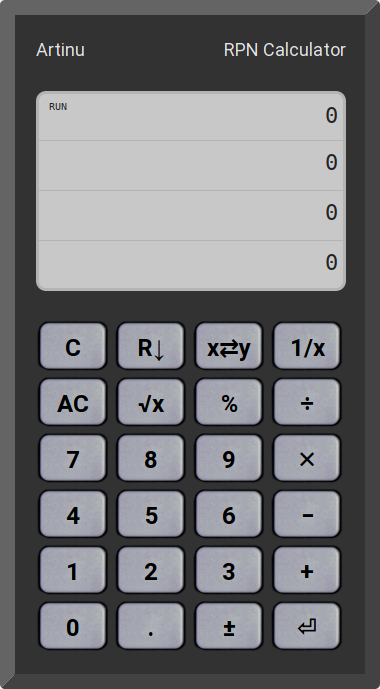# RPN Calculator

Reverse Polish Notation is a method of notation of mathematical expressions that allows simple calculations to be performed without the need of using brackets – thanks to the use of a stack. This method has been popularized by Hewlett Packard, which has been successfully using it in its calculators for many years.

The Artinu application is a simple RPN calculator, written in JavaScript, available online:# COVID-19 Death Rate

Update:
$$d = \frac{N_\mathrm{deaths}}{N_{\mathrm{infected}}} \cdot 100,$$
where $$N_\mathrm{deaths}$$ is number of deaths caused by COVID-19 infection since the beginning of epidemic, $$N_{\mathrm{infected}}$$ is a total number of infected people. For each country, the plot starts at the first date where there are at least 100 infected cases, which is common practice.